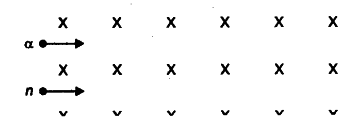# State Ampere's circuital law. Use this law

(a) State Ampere’s circuital law. Use this law to obtain the expression for the magnetic field inside an
air cored toroid of average radius V, having turns per unit length and carrying a steady current I.
(b) An observer to the left of a solenoid of N turns each of cross section area ‘A’ observes that a steady current I in it flows in the clockwise direction. Depict the magnetic field lines due to the solenoid specifying its polarity and show that acts as a bar magnet of magnetic moment m = NIA.(a) Line integral of magnetic field over a dosed loop is equal to the total current passing through the surface enclosed by the loop. Alternatively,